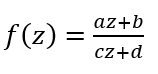## Conformal Maps

Here we examine a different way of understanding analytic functions.  We view them through the lens of geometry instead of analysis.  Recall that the angle between two curves is given by the angle of their tangents lines at the point of intersection.  A conformal map is a map that preserves angles between two intersecting curves.  Consider the curves given by γ1 and γ2 and the analytic function φ(z) = z .

3It may not be readily apparent from the above graphs because of scale differences, but φ maps the right angle in the above left-hand figure to the right angle in the above right-hand figure.

Theorem x: Suppose that φ is analytic in a domain D.  Then φ is conformal on every pair of intersecting curves provided the derivative of φ is nonzero at the point of intersection.

## Linear Fractional Transformations

There are particular conformal maps that we are especially interested in.  Among these

are linear fractional transformations.  We define these functions as given to right.  a, b, c, d are all

complex coefficients (possibly 0).   In general, we require that ad - bc ≠ 0 otherwise f is a constant

function.  These are also sometimes referred to as Moebius transforms or bilinear transforms.

Three points in the form (z1, w1), (z2, w2), and (z3, w3) are                                                                                                              sufficient to define a linear fractional transformation.  A quick                                                                                                        way to calculate a linear fractional transformation is via the                                                                                                          cross ratio shown to the left.  Linear fractional transformations also preserve orientation and take boundaries to boundaries (though they may invert them)!Consider the linear fractional transformation defined by T(i) = 0, T(∞) = 1, and T(-1) = i.  Write T(z) out in the standard form of a linear fraction transformation.